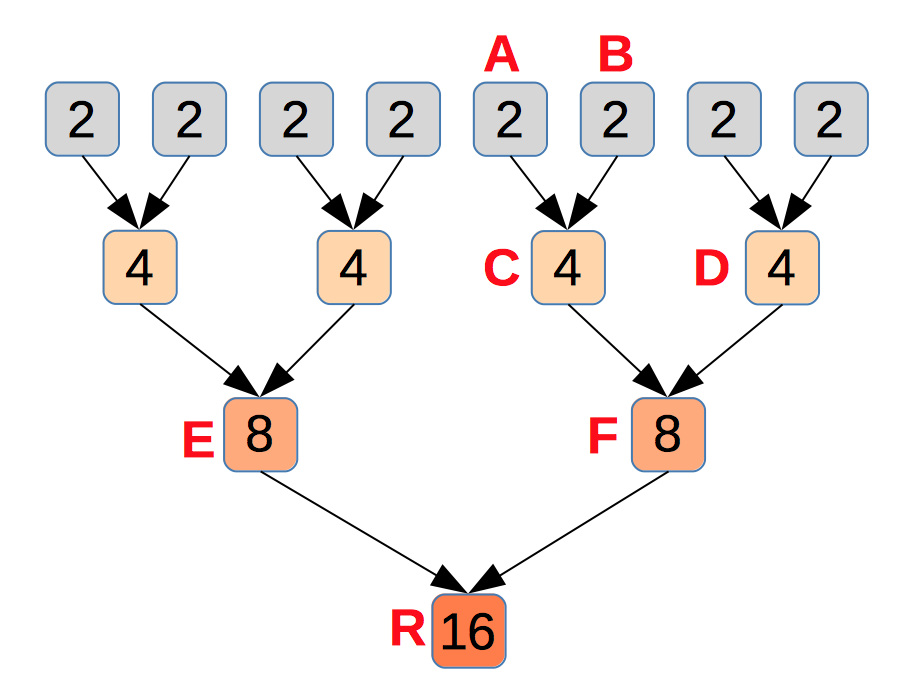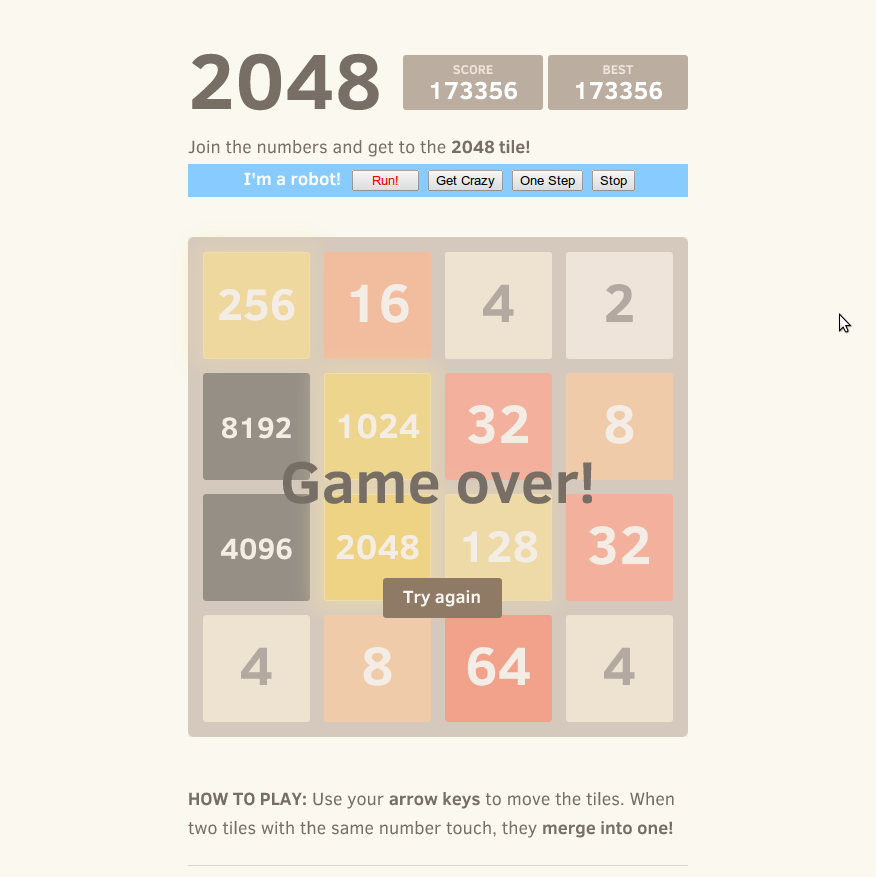01 April 2014

A couple of weeks ago, one intern of my team re-posted a game called 2048 into our wechat group. Soon we found we could not stop playing the game. And some of us started to post screenshots of scores we got in the game. We made higher and higher scores and finally completed 2048 tiles one day later. But we didn’t stop, we kept going and tried to accomplish even bigger tile than 2048.

So I thought about one question:

#### What is the biggest tile that can be made in the game?

We know that two 2s can be merged to make a 4, and two 4s can be merged to make an 8. And eventually, any big tile is made out of basic tiles, i.e. 2s or 4s. And after reading a little bit of code of the game, I found that the basic tiles appearing on the board have 90% probability to be a 2 and 10% to be a 4.

Let’s ignore 4s first. We assume all new tiles are 2s. So any big tile is made from a set of 2s, via a sequence of merges. E.g. a 16 is made out of eight 2s via 7 merges. I drew a tree to illustrate these merges for the 16:The two 2s, tile A and tile B, happen to be the LAST pair of 2s merged in the game. We can always find such a pair of 2s in any tree for a 16 or other big tiles.

Consider the moment that tile A is merged(with B). There is a path from A to the root R: A->C->F->R. All tiles in the path except A don’t exist at that moment. So, look at those tiles going to be merged with a tile in the path, i.e. the brothers of tiles in the path: tile B, D and E. Each of them either exists on the board, or will be made from some tiles existing on the board, because all 2s in the tree except A and B have already been merged and new 2s coming out later will not be used to make the 16. In both cases, there is at least one tile existing on the board for each of these brothers.

Now we can calculate the number of tiles on the board at that moment: A, B, D or its component tiles, and E or its comonent tiles. There are at least 4 tiles. It equals to the length of the path from A to the root! Yes, generally, to make a 2^N, the number of tiles on the board when the last two 2s are going to be merged is at least N – the height of the ‘merge’ tree for 2^N.

So the 2048 game has exactly 16 slots and can not make a 2^17 in any way.

Back to the original 2048 game, a basic tile has 10% possibility to be 4. With some similar analysis, we can know that the game can not make a 2^18 even even if all basic tiles coming out are 4s.

Knowing the biggest possible tile in the game won’t help me play the game better. So I tried to implement a program to run the game for me.

#### Implement a 2048 AI with Javascript

I first wrote a C++ AI program for the game. Since it’s almost impossible to make a 2^16, I encoded a tile in 4 bit and encoded the whole board in a 64bit integer. So I could use bitwise operations to simulate movements easily. But a C++ program can not run in browser. And it’s not cool if it can not be put onto the web. So I rewrote it with javascript.

I had not written any javascript code to solve such a problem requiring so much computing. I had planed to search at least 10 steps and use some kind of cache map to avoid duplicated computing. But unfortunately I found javascript is far slower than C++. So I decided to only search 3 steps in most cases so that I can keep the amount of computing in a reasonable range.

I used javascript objects as maps to cache searching results and avoid duplicated computing before. But later I found it actually hurt the performance. I removed all of these code and made the program run twice as fast as before.

After some optimizations, I finally made the AI program run pretty fast. On the chrome browser in my laptop, it usually spends less than 30 seconds to make a 2048. And it hardly fails to make a 2048 and often successfully makes a 4096 or 8192. And I have even made a 16384!#### The AI algorithm

The code has is quite short. The basic idea is to find the direction for each movement on which the game can get the maximal expected score. It’s expected score because a new tile can appear at any empty slot with the same possibility, and has 90% chance to be 2 and 10% to be 4.

The algorithm is:

``````Search(grid, step) {
if (step == max_step)
return Estimate(grid);
best_score = -INFINITE;
for (i = 0; i < 4; ++i) {
[new_grid, score] = MoveLeft(grid);
if (new_grid == grid) continue;
k = CountEmptySlot(new_grid);
if (k == 0) {
score += HUGE_PENALTY;
} else {
for (j = 0; j < 16; ++j) {
if (new_grid[j] is empty) {
new_grid[j] = 2;
score += 1 / k * 0.9 * Search(new_grid, step+1);
new_grid[j] = 4;
score += 1 / k * 0.1 * Search(new_grid, step+1);
reset new_grid[j];
}
}
}
if (score > best_score) best_score = score;
new_grid = Rotate(new_grid);
}
return best_score;
}``````

Estimate() is to estimate the state after max_step movements. I tried a couple of functions and found a pretty good function. In the function, the estimation score will be punished by the differences of adjacent tiles. So states with high estimation scores usually put the biggest tile at one of the four corners because the number of adjacent tiles is mimimum if at corner, and put other big tiles at the edge of the board and adjacent to a bigger tile.

``````Estimate(grid) {
sum = 0
penalty = 0
for (i = 0; i < 16; ++i) {
sum += grid[i];
if (i % 4 != 3) {
penalty += Math.abs(grid[i] - grid[i + 1]);
}
if (i < 12) {
penalty += Math.abs(grid[i] - grid[i + 4]);
}
}
return (sum * 4 - penalty) * 2;
}``````

I use a very large penalty(-10^20) for a dead state. So the program will try its best to avoid dead states. Although it maybe over evaluates dead states, I want the program to avoid the risk of failing before making a 2048.

By default, the max_step is 3. If when a search ends the number of visited states is less than 10000, it will start a new search with max_step increased by 1. So the program will keep searching more and more deeply until the number of visited states is larger than 10000. Usually there are much fewer states can be reached in a bad situation than a good situation. The strategy can make sure the program does enough searchs when the game goes bad and doesn’t waste too much time when the game goes well. There are indeed some duplicated computing to start a completely new search. But since the number of visited states increases approximately exponentially with the depth, only a small portion of computing is duplicated.

The game I forked and added AI into is in: http://sleepycoder.github.io/2048/. The AI code is in http://sleepycoder.github.io/2048/ai.js. And I also made a chrome extension for the AI.

In the end, I posted a 70,000+ and later a 170,000+ in the group and successfully extinguished others’ passions on the game:)